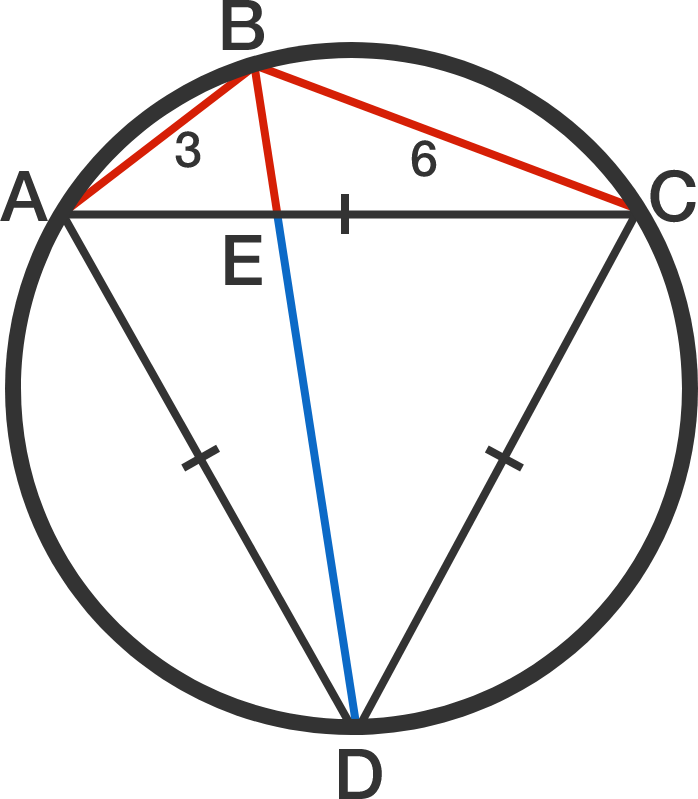# Ptolemy's Riddle IIIA cyclic quadrilateral $ABCD$ is constructed within a circle such that $AB = 3, BC = 6,$ and $\triangle ACD$ is equilateral, as shown to the right.

If $E$ is the intersection point of both diagonals of $ABCD$, what is the length of $ED,$ the blue line segment in the diagram?

×